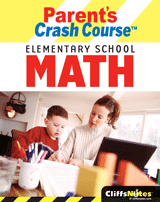# CliffsNotes Parent's Crash Course: Elementary School Math

by David A Herzog
\$9.99
1

• Format: eBook
• ISBN-13/EAN: 9780544183193
• ISBN-10: 0544183193
• Pages: 266
• Publication Date: 05/03/2007

Is helping your kids with elementary math homework a problem?
* 6,234 + 5,893 + 475 + 872 =
* What is the greatest common factor for 140 and 175?
* Find the percentage: 25,000 cheering for the home team in an arena holding 40,000 fans
* (8) + (-7) + (12) + (-11) + (15) + (-9) =
* Express 343 in terms of its simplest base and exponent form.

(See answers at bottom of page)

It's probably been awhile since you took math, and math is taught differently today. So when your kids need help with the dreaded math homework, you may be pressed to remember some of the basics in addition to being pressed for time!

CliffsNotes Parent's Crash Course Elementary School Math is the answer! This one-of-a-kind book features:
* Practical organization that helps you get up to speed quickly
* 58 different lessons explained in two- to three-page units
* Quick explanations of essential terms at the beginning of each chapter
* Factoids that get your kids more interested
* Exercises and answers at the end of many lessons
* An appendix containing flashcards for added practice

The book covers math concepts and principles taught in elementary school, including:
* Whole numbers
* Decimals
* Fractions
* Percents
* Integers
* Powers, exponents, and roots
* Powers of ten
* Measurements
* Geometry
* Multiplying and dividing